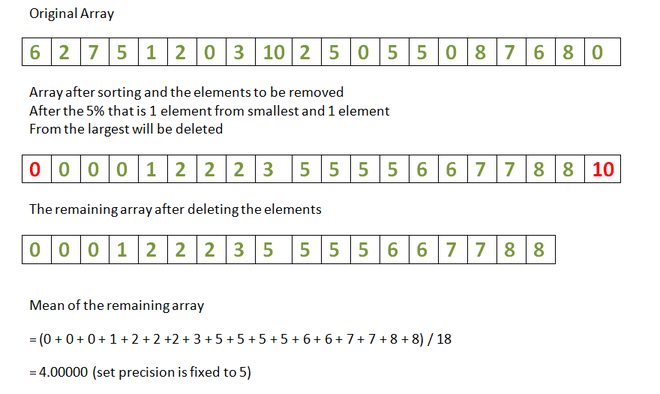Mean of given array after removal of K percent of smallest and largest array elements

• Last Updated : 12 May, 2021

Given an array arr[] and an integer K, the task is to remove K % percent array elements from the smallest and largest array elements and calculate the mean of the remaining array.

Examples:

Input: arr[] = {6, 2, 7, 5, 1, 2, 0, 3, 10, 2, 5, 0, 5, 5, 0, 8, 7, 6, 8, 0}, K = 5
Output: 4.00000
Explanation:
There are 20 elements in the array. Therefore, 5% of 20 is 1. Therefore, 1 of the smallest elements (i.e. 0) is removed and 1 element of the largest elements (i.e. 0) is removed. Therefore, mean of the remaining array is 18.Input: arr[] = {6, 0, 7, 0, 7, 5, 7, 8, 3, 4, 0, 7, 8, 1, 6, 8, 1, 1, 2, 4}, K = 10
Output: 4.31250

Approach:

1. Sort the array arr[].
2. Find the size of the array.
3. Calculate the K-th percent of the size of the array.
4. Now, add the elements present in the indices K% to (N – 1) – K%.
5. Finally, find the mean of those elements.

Below is the implementation of the above approach:

C++14

 // C++ program for the above approach#include using namespace std; // Function to calculate the mean// of a given array after removal// of Kth percent of smallest and// largest array elementsvoid meanOfRemainingElements(int arr[],                             int N, int K){    // Sort the array    sort(arr, arr + N);     // Find the K-th percent    // of the array size    int kthPercent = (N * K) / 100;    float sum = 0;     // Traverse the array    for (int i = 0; i < N; i++)         // Skip the first K-th        // percent & last K-th        // percent array elements        if (i >= kthPercent && i < (N - kthPercent))            sum += arr[i];     // Mean of the rest of elements    float mean = sum                 / (N - 2 * kthPercent);     // Print mean upto 5 decimal places    cout << fixed << setprecision(5) << mean << endl;} // Driver Codeint main(){     int arr[] = { 6, 2, 7, 5, 1, 2, 0, 3, 10, 2,                  5, 0, 5, 5, 0, 8, 7, 6, 8, 0 };    int arr_size = sizeof(arr) / sizeof(arr);    int K = 5;     meanOfRemainingElements(arr, arr_size, K);     return 0;}

Java

 // Java program for the above approachimport java.util.*;public class GFG{   // Function to calculate the mean  // of a given array after removal  // of Kth percent of smallest and  // largest array elements  static void meanOfRemainingElements(int[] arr, int N,                                      int K)  {     // Sort the array    Arrays.sort(arr);     // Find the K-th percent    // of the array size    int kthPercent = (N * K) / 100;    float sum = 0f;     // Traverse the array    for (int i = 0; i < N; i++)       // Skip the first K-th      // percent & last K-th      // percent array elements      if (i >= kthPercent && i < (N - kthPercent))        sum += arr[i];     // Mean of the rest of elements    float mean = (sum / (N - 2 * kthPercent));     // Print mean upto 5 decimal places    System.out.format("%.5f", mean);  }    // Driver Code  public static void main(String args[])  {    int[] arr = { 6, 2, 7, 5, 1, 2, 0, 3, 10, 2,                 5, 0, 5, 5, 0, 8, 7, 6, 8,  0 };    int arr_size = arr.length;    int K = 5;     meanOfRemainingElements(arr, arr_size, K);  }} // This code is contributed by jana_sayantan.

Python3

 # Python program for the above approach # Function to calculate the mean# of a given array after removal# of Kth percent of smallest and# largest array elementsdef meanOfRemainingElements(arr, N, K):       # Sort the array    arr.sort()     # Find the K-th percent    # of the array size    kthPercent = (N * K) / 100    sum = 0     # Traverse the array    for i in range(N):         # Skip the first K-th        # percent & last K-th        # percent array elements        if (i >= kthPercent and i < (N - kthPercent)):            sum += arr[i]     # Mean of the rest of elements    mean = sum/ (N - 2 * kthPercent)     # Print mean upto 5 decimal places    print( '%.5f'%mean) # Driver Codearr = [ 6, 2, 7, 5, 1, 2, 0, 3, 10, 2, 5, 0, 5, 5, 0, 8, 7, 6, 8, 0 ]arr_size = len(arr)K = 5 meanOfRemainingElements(arr, arr_size, K) # This code is contributed by rohitsingh07052.

C#

 // C# program for the above approachusing System; class GFG {     // Function to calculate the mean    // of a given array after removal    // of Kth percent of smallest and    // largest array elements    static void meanOfRemainingElements(int[] arr, int N,                                        int K)    {               // Sort the array        Array.Sort(arr);         // Find the K-th percent        // of the array size        int kthPercent = (N * K) / 100;        float sum = 0f;         // Traverse the array        for (int i = 0; i < N; i++)             // Skip the first K-th            // percent & last K-th            // percent array elements            if (i >= kthPercent && i < (N - kthPercent))                sum += arr[i];         // Mean of the rest of elements        float mean = (sum / (N - 2 * kthPercent));         // Print mean upto 5 decimal places        Console.WriteLine(Math.Round(mean,5));    }     // Driver Code    public static void Main()    {        int[] arr = { 6, 2, 7, 5, 1, 2, 0, 3, 10, 2,                      5, 0, 5, 5, 0, 8, 7, 6, 8,  0 };        int arr_size = arr.Length;        int K = 5;         meanOfRemainingElements(arr, arr_size, K);    }} // This code is contributed by chitranayal.

Javascript



Output:
4.00000

Time Complexity: O(N * logN)
Auxiliary Space: O(N)

My Personal Notes arrow_drop_up